## Tag: computational

#### Fifty Solitaires – The Upgrade

It has been well over a year since I last posted about this project. I have been busy with other projects and had to leave this one on the back burner. A lot has changed since then and the first thing that I noticed when looking at this project again was that I now needed to upgrade all the project’s dependencies to their latest versions. This might not be very interesting from a software development perspective, but it is a necessary step to ensure that the project is up to date. So, this post will be all about upgrading dependencies, making adjustments to the configuration and fixing any issues that might arise.

### Attempting to run the last version of the project

Before I started upgrading dependencies, I wanted to make sure that the project still worked as expected. So, I cloned the repository and ran the following commands.

npm install

Right away, I got error messages from node-gyp.js when it was trying to build the binaries of node-sass. I have seen this error before and it is usually caused by the fact that the version of node-sass is not compatible with the version of Node.js that I am using. So, I checked that I currently have Node.js version 20.5.1 installed and then I checked the node-sass documentation to see that I need version 9.0.0 of node-sass. So, I updated the package.json file to use the current latest version.

"node-sass": "^9.0.0",

Remember that I had already created some components and stories for Storybook, but I had not yet implemented the main application. So, I ran Storybook to see if the components would still show up as expected.

npm run storybook

Storybook uses Webpack 4 to bundle the project. This emitted the following error message

Error: error:0308010C:digital envelope routines::unsupported

Again, this is a compatibility problem with Node. On StackOverflow, I found a solution that suggested telling Node.js to enable the legacy openssl provider. This can be done by setting an environment variable.

export NODE_OPTIONS=--openssl-legacy-provider
npm run storybook

This time, Storybook started up and I could see the project in the browser. At this point, I am hoping this environment variable will not be needed once I have upgraded all the dependencies. But for now, I will leave it in place.

Now that I can see the Card and Pile components in Storybook, I will start upgrading the dependencies. I had two options. Either I could try to update one dependency at a time and then run Storybook each time to see if it still worked. Or I could update all the dependencies at once and then fix any issues that might arise. The problem with the first approach is that some upgrades might not be independent of each other. This could create more work, trying to figure out all the errors in the intermediate stages that wouldn’t have occurred if I had just upgraded everything at once. So, I decided to go with the second approach. To do this, I removed all entries  dependencies from the package.json file. I made a note of all the packages so that I could reinstall them later.

"dependencies": {},

To reinstall all the packages, I first removed the node_modules folder and the package-lock.json file. Then I ran npm install with all the packages I had noted down from the dependencies section of the package.json file.

rm -rf node_modules package-lock.json
npm install @testing-library/jest-dom @testing-library/react \
@testing-library/user-event @types/jest @types/node @types/react \
@types/react-dom react node-sass react-dom react-scripts \
typescript web-vitals

Note, that I did not touch the devDependencies entry in packages.json. Looking at those entries, I saw that devDependencies only contains packages related to Storybook. This will be upgraded in a separate step. To test that the React application was still working, I ran the following command.

npm start

This worked fine. Of course, the application is only showing the default React page. Previously, I had not yet added anything to the front page because I was first focusing on creating the Card and Pile components and their Storybook stories. So, now it was time to upgrade Storybook. Fortunately, Storybook provides a command that can perform this task for you. From the documentation, I found that I should run the following command to upgrade Storybook.

npx storybook@latest upgrade

This prompted me with several questions about which migrations to run. I answered y to all of them. This step completed without any problems.

### Fixing Remaining Issues

Now, it was time to check if the upgrade of Storybook had caused any issues. I ran the following command.

npm run storybook

Storybook starts but then crashes. In the browser, I could only see the spinner. In the terminal, I was getting an extremely long error message that contained the full contents of my playing-cards.svg file. At the top of the error message, I see the following information.

SyntaxError: unknown: Namespace tags are not supported by default. React's JSX doesn't support namespace tags. 

Putting this error message into Google helped. This StackOverflow post helped me figure it out. My SVG file contains quite a few namespace tags but React does not understand namespace tags. By renaming them with a camel-cased variant I was able to get rid of the error. This was a bit tedious, but it did the trick. I am not quite sure why this was working before, but I am glad I was able to fix it.

### Final Thoughts

After completing all the steps above, Storybook worked again. To double-check, I also opened a new terminal and tested Storybook without setting the NODE_OPTIONS environment variable. This means the intermediate fix for the legacy openssl provider was no longer necessary.

In conclusion, I was dreading the upgrade of the project’s dependencies. But apart from a few glitches, it turned out to be not too hard. In a previous attempt, I did not use the Storybook command and, instead, tried to upgrade Storybook manually. This did not work out so well. It shows how important it is to spend some time studying the documentation of the tools that you are using.

#### Computational Physics Basics: Polynomial Interpolation

The piecewise constant interpolation and the linear interpolation seen in the previous post can be understood as special cases of a more general interpolation method. Piecewise constant interpolation constructs a polynomial of order 0 that passes through a single point. Linear interpolation constructs a polynomial of order 1 that passes through 2 points. We can generalise this idea to construct a polynomial of order $$n-1$$ that passes through $$n$$ points, where $$n$$ is 1 or greater. The idea is that a higher-order polynomial will be better at approximating the exact function. We will see later that this idea is only justified in certain cases and that higher-order interpolations can actually increase the error when not applied with care.

### Existence

The first question that arises is the following. Given a set of $$n$$ points, is there always a polynomial of order $$n-1$$ that passes through these points, or are there multiple polynomials with that quality? The first question can be answered simply by constructing a polynomial. The simplest way to do this is to construct the Lagrange Polynomial. Assume we are given a set of points, $(x_1, y_1), (x_2, y_2) \ldots (x_n, y_n),$
where all the $$x$$’s are different, i.e. $$x_i \ne x_j$$ if $$i \ne j$$. Then we observe that the fraction $\frac{x – x_j}{x_i – x_j}$
is zero when $$x = x_j$$ and one when $$x = x_i$$. Next, let’s choose an index $$i$$ and multiply these fractions together for all $$j$$ that are different to $$i$$, $a_i(x) = \frac{x – x_1}{x_i – x_1}\times \ldots\times\frac{x – x_{i-1}}{x_i – x_{i-1}} \frac{x – x_{i+1}}{x_i – x_{i+1}}\times \ldots\times\frac{x – x_n}{x_i – x_n}.$
This product can be written a bit more concisely as $a_i(x) = \prod_{\stackrel{j=1}{j\ne i}}^n \frac{x – x_j}{x_i – x_j}.$
You can see that the $$a_i$$ are polynomials of order $$n-1$$. Now, if $$x = x_i$$ all the factors in the product are 1 which means that $$a_i(x_i) = 1$$. On the other hand, if $$x$$ is any of the other $$x_j$$ then one of the factors will be zero and $$a_i(x_j) = 0$$ for any $$j \ne i$$. Thus, if we take the product $$a_i(x) y_i$$ we have a polynomial that passes through the point $$(x_i, y_i)$$ but is zero at all the other $$x_j$$. The final step is to add up all these separate polynomials to construct the Lagrange Polynomial, $p(x) = a_1(x)y_1 + \ldots a_n(x)y_n = \sum_{i=1}^n a_i(x)y_i.$
By construction, this polynomial of order $$n-1$$ passes through all the points $$(x_i, y_i)$$.

### Uniqueness

The next question is if there are other polynomials that pass through all the points, or is the Lagrange Polynomial the only one? The answer is that there is exactly one polynomial of order $$n$$ that passes through $$n$$ given points. This follows directly from the fundamental theorem of algebra. Imagine we have two order $$n-1$$ polynomials, $$p_1$$ and $$p_2$$, that both pass through our $$n$$ points. Then the difference, $d(x) = p_1(x) – p_2(x),$
will also be an order $$n-1$$ degree polynomial. But $$d$$ also has $$n$$ roots because $$d(x_i) = 0$$ for all $$i$$. But the fundamental theorem of algebra asserts that a polynomial of degree $$n$$ can have at most $$n$$ real roots unless it is identically zero. In our case $$d$$ is of order $$n-1$$ and should only have $$n-1$$ roots. The fact that it has $$n$$ roots means that $$d \equiv 0$$. This in turn means that $$p_1 = p_2$$ must be the same polynomial.

### Approximation Error and Runge’s Phenomenon

One would expect that the higher order interpolations will reduce the error of the approximation and that it would always be best to use the highest possible order. One can find the upper bounds of the error using a similar approach that I used in the previous post on linear interpolation. I will not show the proof here, because it is a bit more tedious and doesn’t give any deeper insights. Given a function $$f(x)$$ over an interval $$a\le x \le b$$ and sampled at $$n+1$$ equidistant points $$x_i = a + hi$$, with $$i=0, \ldots , n+1$$ and $$h = (b-a)/n$$, then the order $$n$$ Lagrange polynomial that passes through the points will have an error given by the following formula. $\left|R_n(x)\right| \leq \frac{h^{n+1}}{4(n+1)} \left|f^{(n+1)}(x)\right|_{\mathrm{max}}$
Here $$f^{(n+1)}(x)$$ means the $$(n+1)$$th derivative of the the function $$f$$ and the $$\left|.\right|_{\mathrm{max}}$$ means the maximum value over the interval between $$a$$ and $$b$$. As expected, the error is proportional to $$h^{n+1}$$. At first sight, this implies that increasing the number of points, and thus reducing $$h$$ while at the same time increasing $$n$$ will reduce the error. The problem arises, however, for some functions $$f$$ whose $$n$$-th derivatives grow with $$n$$. The example put forward by Runge is the function $f(x) = \frac{1}{1+25x^2}.$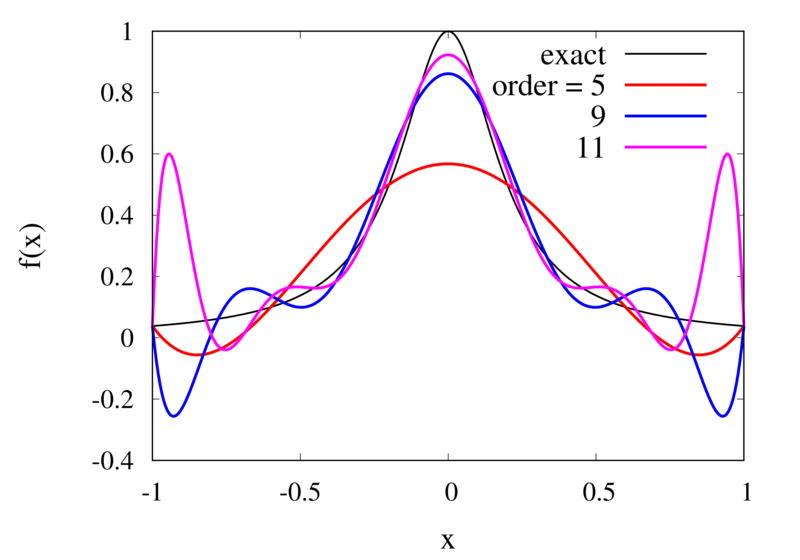Interpolation of Runge’s function using higher-order polynomials.

The figure above shows the Lagrange polynomials approximating Runge’s function over the interval from -1 to 1 for some orders. You can immediately see that the approximations tend to improve in the central part as the order increases. But near the outermost points, the Lagrange polynomials oscillate more and more wildly as the number of points is increased. The conclusion is that one has to be careful when increasing the interpolation order because spurious oscillations may actually degrade the approximation.

### Piecewise Polynomial Interpolation

Does this mean we are stuck and that moving to higher orders is generally bad? No, we can make use of higher-order interpolations but we have to be careful. Note, that the polynomial interpolation does get better in the central region when we decrease the spacing between the points. When we used piecewise linear of constant interpolation, we chose the points that were used for the interpolation based on where we wanted to interpolate the function. In the same way, we can choose the points through which we construct the polynomial so that they are always symmetric around $$x$$. Some plots of this piecewise polynomial interpolation are shown in the plot below.Piecewise Lagrange interpolation with 20 points for orders 0, 1, 2, and 3.

Let’s analyse the error of these approximations. Using an array with $$N$$ points on Runge’s function equally spaced between -2 and 2. $$N$$ was varied between 10 and 10,000. For each $$N$$, the centred polynomial interpolation of orders 0, 1, 2, and 3 was created. Finally, the maximum error of the interpolation and the exact function over the interval -1 and 1 are determined.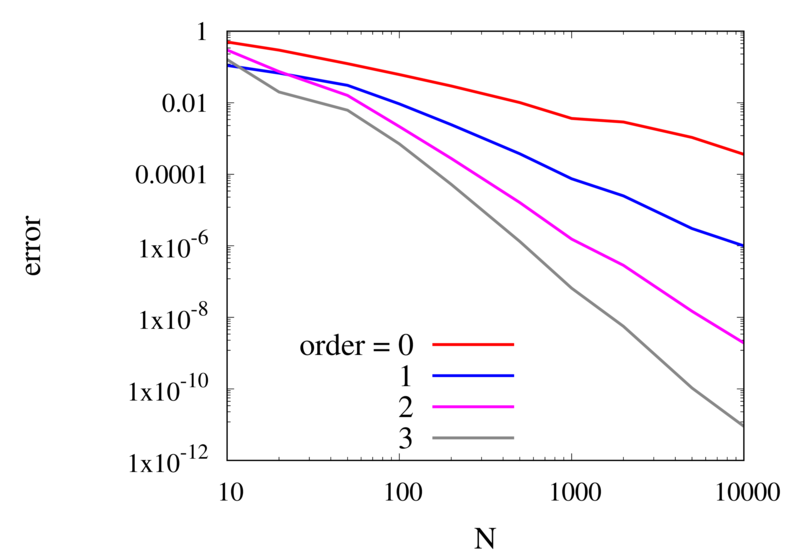Scaling of the maximum error of the Lagrange interpolation with the number of points for increasing order.

The plot above shows the double-logarithmic dependence of the error against the number of points for each order interpolation. The slope of each curve corresponds to the order of the interpolation. For the piecewise constant interpolation, an increase in the number of points by 3 orders of magnitude also corresponds to a reduction of the error by three orders of magnitude. This indicates that the error is first order in this case. For the highest order interpolation and 10,000 points, the error reaches the rounding error of double precision.

### Discontinuities and Differentiability

As seen in the previous section, for many cases the piecewise polynomial interpolation can provide a good approximation to the underlying function. However, in some cases, we need to use the first or second derivative of our interpolation. In these cases, the Lagrange formula is not ideal. To see this, the following image shows the interpolation error, again for Runge’s function, using order 2 and 3 polynomials and 20 points.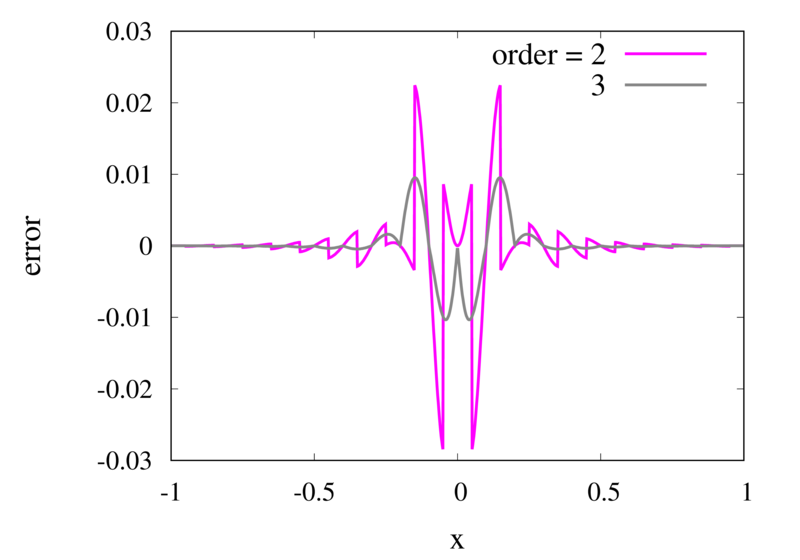Error in the Lagrange interpolation of Runge’s function for orders 2 and 3.

One can see that the error in the order 2 approximation has discontinuities and the error in the order 3 approximation has discontinuities of the derivative. For odd-order interpolations, the points that are used for the interpolation change when $$x$$ moves from an interval $$[x_{i-1},x_i]$$ to an interval $$[x_i, x_{i+1}]$$. Because both interpolations are the same at the point $$x_i$$ itself, the interpolation is continuous but the derivative, in general, is not. For even-order interpolations, the stencil changes halfway between the points, which means that the function is discontinuous there. I will address this problem in a future post.

#### Starting GPU programming with Kokkos

The high performance computing landscape has changed quite a bit over the last years. For quite a few decades, the major architecture for HPC systems was based on classical CPU processors distributed over many nodes. The nodes are connected via a fast network architecture. These compute clusters have evolved over time. A typical simulation code that makes use of this architecture will consist of more or less serial code that runs on each core and communicates with the other cores via the message passing library MPI. This has the drawback that the serial instances of the simulation code running on a shared memory node still have to exchange data through this mechanism, which takes time and reduces efficiency. On many current systems, each node can have on the order of 10s of compute cores accessing the same shared memory. To make better use of these cores, multithreading approaches, such as OpenMP, have been used increasingly over the past decade or so. However, there are not many large scale simulation codes that make use of OpenMP and MPI at the same time to reach maximum performance.

More recently, there has been new style of architecture that has become a serious player. GPU processors that used to play more of an outsider role in serious high performance computing have taken centre stage. Within the DoE’s Exascale project in the US, new supercomputers are being built that are breaking the exaflop barrier. These machines rely on a combination of traditional CPU cluster with GPU accelerators.

For developers of high performance simulation codes this means that much of their code has to be re-written to make use of the new mixed architectures. I have been developing the Schnek library for a number of years now and I am using it form many of my simulation codes. To keep the library relevant for the future, I am currently in the process of adding mixed architecture capabilities to Schnek. Fortunately, there already exists the Kokkos library which provides an abstraction layer over the different machine architectures. It also provides multi-dimensional arrays that can be stored on the host memory or on the accelerator’s device memory. It also provides iteration mechanisms to process these arrays on the CPU or GPU.

In this post, I am describing my first experiences with Kokkos and how it can be used to accelerate calculations. I will be starting from scratch which means that I will begin by installing Kokkos. The best way to do this nowadays is to use a packet manager called Spack. Spack is designed as a packet manager for supercompters but will work on any regular Linux machine. To start, I navigate into a folder where I want to install spack, let’s call it /my/installation/folder. I then simply clone Spack’s git repository.

git clone -c feature.manyFiles=true https://github.com/spack/spack.git

I am using the bash shell, so now I can initialise all the environment variables by running

source /my/installation/folder/spack/share/spack/setup-env.sh

This command should be run every time you want to start developing code using the packages supplied by Spack. I recommend not putting this line into your .bashrc because some of the Spack packages might interfere with your regular system operation.

The Kokkos package has many configuration options. You can now look at them using the command

spack info kokkos

I have an NVidia Quadro P1000 graphics card on my local development machine. For NVidia accelerators, I will need to install Kokkos with Cuda support. In order to install the correct Cuda version, I first check on https://developer.nvidia.com/cuda-gpus for the compute capability. For my hardware, I find that I will need version 6.1. The following command will install Kokkos and all of its dependencies.

spack install kokkos +cuda +wrapper cuda_arch=61

The wrapper option is required because I am compiling everything with the gcc compiler. Note that this can take quite some time because many of the packages may be build from source code during the installation. This allows Spack packages to be highly specific to your system.

spack load kokkos

Now, I can start creating my first Kokkos example. I create a file called testkokkos.cpp and add some imports to the top.

#include <Kokkos_Core.hpp>
#include <chrono>

The Kokkos_Core.hpp import is needed to use Kokkos and I also included chrono from the standard library to allow timing the code. Kokkos introduces execution spaces and memory spaces. Data can live on the host machine or the accelerator device. Similarly, execution of the code can be carried out on the serial CPU or the GPU or some other specialised processor. I want my code to be compiled for two different settings so that I can compare GPU performance against the CPU. I define two types Execution and Memory for the execution and memory spaces. These types will depend on an external macro that will be passed in by the build system.

#ifdef TEST_USE_GPU
typedef Kokkos::Cuda Execution;
typedef Kokkos::CudaSpace Memory;
#else
typedef Kokkos::Serial Execution;
typedef Kokkos::HostSpace Memory;
#endif

Kokkos manages data in View objects which represent multi-dimensional arrays. View has some template arguments. The first argument specifies the dimensionality and the datatype stored in the array. Further template arguments can be specified to specify the memory space and other compile-time configurations. For example Kokkos::View<double**, Kokkos::HostSpace> defines a two dimensional array of double precision values on the host memory. To iterate over a view, one needs to define function objects that will be passed to a function that Kokkos calls “parallel dispatch”. The following code defines three such structs that can be used to instantiate function objects.

#define HALF_SIZE 500

struct Set {
Kokkos::View<double**, Memory> in;

KOKKOS_INLINE_FUNCTION void operator()(int i, int j) const {
in(i, j) = i==HALF_SIZE && j==HALF_SIZE ? 1.0 : 0.0;
}
};

struct Diffuse {
Kokkos::View<double**, Memory> in;
Kokkos::View<double**, Memory> out;

KOKKOS_INLINE_FUNCTION void operator()(int i, int j) const {
out(i, j) = in(i, j) + 0.1*(in(i-1, j) + in(i+1, j) + in(i, j-1) + in(i, j+1)  - 4.0*in(i, j));
}
};

The Set struct will initialise an array to 0.0 everywhere except for one position where it will be set to 1.0. This results in a single spike in the centre of the domain. Diffuse applies a diffusion operator to the in array and stored the result in the out array. The calculations can’t be carried out in-place because the order in which the function objects are called may be arbitrary. This means that, after the diffusion operator has been applied, the values should be copied back from the out array to the in array.

Now that these function objects are defined, I can start writing the actual calculation.

void performCalculation() {
const int N = 2*HALF_SIZE + 1;
const int iter = 100;

Kokkos::View<double**, Memory> in("in", N, N);
Kokkos::View<double**, Memory> out("out", N, N);

Set set{in};
Diffuse diffuse{in, out};

The first two line in the function define some constants. N is the size of the grids and iter sets the number of times that the diffusion operator will be applied. The Kokkos::View objects in and out store the 2-dimensional grids. The first template argument double ** specifies that the arrays are 2-dimensional and store double values. The Memory template argument was defined above and can either be Kokkos::CudaSpace or Kokkos::HostSpace. The last two lines in the code segment above initialise my two function objects of type Set and Diffuse.

I want to iterate over the inner domain, excluding the grid points at the edge of the arrays. This is necessary because the diffusion operator accesses the grid cells next to the position that is iterated over. The iteration policy uses the multidmensional range policy from Kokkos.

    auto policy = Kokkos::MDRangePolicy<Execution, Kokkos::Rank<2>>({1, 1}, {N-1, N-1});

The Execution template argument was defined above and can either be Kokkos::Cuda or Kokkos::Serial. The main calculation now looks like this.

    Kokkos::parallel_for("Set", policy, set);
Kokkos::fence();

auto startTime = std::chrono::high_resolution_clock::now();

for (int i=0; i<iter; ++i)
{
Kokkos::parallel_for("Diffuse", policy, diffuse);
Kokkos::fence();
Kokkos::deep_copy(in,  out);
}

auto endTime = std::chrono::high_resolution_clock::now();
auto milliseconds = std::chrono::duration_cast<std::chrono::milliseconds>(endTime - startTime).count();

std::cerr << "Wall clock: " <<  milliseconds << " ms" << std::endl;
}

The function Kokkos::parallel_for applies the function object for each element given by the iteration policy. Depending on the execution space, the calculations are performed on the CPU or the GPU. To set up the calculation the set operator is applied. Inside the main loop, the diffusion operator is applied, followed by a Kokkos::deep_copy which copies the out array back to the in array. Notice, that I surrounded the loop by calls to STL’s high_resolution_clock::now(). This will allow me to print out the wall-clock time used by the calculation and give me some indication of the performance of the code.

The main function now looks like this.

int main(int argc, char **argv) {
Kokkos::initialize(argc, argv);

performCalculation();

Kokkos::finalize_all();
return 0;
}

It is important to initialise Kokkos before any calls to its routines are called, and also to finalise it before the program is exited.

To compile the code, I use CMake. Kokkos provides the necessary CMake configuration files and Spack sets all the paths so that Kokkos is easily found. My CMakeLists.txt file looks like this.

cmake_minimum_required(VERSION 3.10)

project(testkokkos LANGUAGES CXX)

set(CMAKE_CXX_STANDARD 17)
set(CMAKE_CXX_STANDARD_REQUIRED ON)
set(CMAKE_CXX_EXTENSIONS OFF)

set(CMAKE_ARCHIVE_OUTPUT_DIRECTORY ${CMAKE_BINARY_DIR}/lib) set(CMAKE_LIBRARY_OUTPUT_DIRECTORY${CMAKE_BINARY_DIR}/lib)
set(CMAKE_RUNTIME_OUTPUT_DIRECTORY ${CMAKE_BINARY_DIR}/bin) find_package(Kokkos REQUIRED PATHS${KOKKOS_DIR})
# find_package(CUDA REQUIRED)

target_compile_definitions(testkokkosCPU PRIVATE KOKKOS_DEPENDENCE)

target_compile_definitions(testkokkosGPU PRIVATE TEST_USE_GPU KOKKOS_DEPENDENCE)
target_link_libraries(testkokkosGPU PRIVATE Kokkos::kokkoscore)

Running cmake . followed by make will build two targets, testkokkosCPU and testkokkosGPU. In the compilation of testkokkosGPU the TEST_USE_GPU macro has been set so that it will make use of the Cuda memory and execution spaces.

Now its time to compare. Running testkokkosCPU writes out

Wall clock: 9055 ms

Running it on the GPU with testkokkosGPU gives me

Wall clock: 57 ms

That’s right! On my NVidia Quadro P1000, the GPU accelerated code outperforms the serial version by a factor of more than 150.

Using Kokkos is a relatively easy and very flexible way to make use of GPUs and other accelerator architectures. As a developer of simulation codes, the use of function operators may seem a bit strange at first. From a computer science perspective, on the other hand, these operators feel like a very natural way of approaching the problem.

My next task now is to integrate the Kokkos approach with Schnek’s multidimensional grids and fields. Fortunately, Schnek provides template arguments that allow fine control over the memory model and this should allow replacing Schnek’s default memory model with Kokkos views.

#### Computational Physics Basics: Piecewise and Linear Interpolation

One of the main challenges of computational physics is the problem of representing continuous functions in time and space using the finite resources supplied by the computer. A mathematical function of one or more continuous variables naturally has an infinite number of degrees of freedom. These need to be reduced in some manner to be stored in the finite memory available. Maybe the most intuitive way of achieving this goal is by sampling the function at a discrete set of points. We can store the values of the function as a lookup table in memory. It is then straightforward to retrieve the values at the sampling points. However, in many cases, the function values at arbitrary points between the sampling points are needed. It is then necessary to interpolate the function from the given data.

Apart from the interpolation problem, the pointwise discretisation of a function raises another problem. In some cases, the domain over which the function is required is not known in advance. The computer only stores a finite set of points and these points can cover only a finite domain. Extrapolation can be used if the asymptotic behaviour of the function is known. Also, clever spacing of the sample points or transformations of the domain can aid in improving the accuracy of the interpolated and extrapolated function values.

In this post, I will be talking about the interpolation of functions in a single variable. Functions with a higher-dimensional domain will be the subject of a future post.

### Functions of a single variable

A function of a single variable, $$f(x)$$, can be discretised by specifying the function values at sample locations $$x_i$$, where $$i=1 \ldots N$$. For now, we don’t require these locations to be evenly spaced but I will assume that they are sorted. This means that $$x_i < x_{i+1}$$ for all $$i$$. Let’s define the function values, $$y_i$$, as $y_i = f(x_i).$
The intuitive idea behind this discretisation is that the function values can be thought of as a number of measurements. The $$y_i$$ provide incomplete information about the function. To reconstruct the function over a continuous domain an interpolation scheme needs to be specified.

#### Piecewise Constant Interpolation

The simplest interpolation scheme is the piecewise constant interpolation, also known as the nearest neighbour interpolation. Given a location $$x$$ the goal is to find a value of $$i$$ such that $|x-x_i| \le |x-x_j| \quad \text{for all} \quad j\ne i.$
In other words, $$x_i$$ is the sample location that is closest to $$x$$ when compared to the other sample locations. Then, define the interpolation function $$p_0$$ as $p_0(x) = f(x_i)$
with $$x_i$$ as defined above. The value of the interpolation is simply the value of the sampled function at the sample point closest to $$x$$.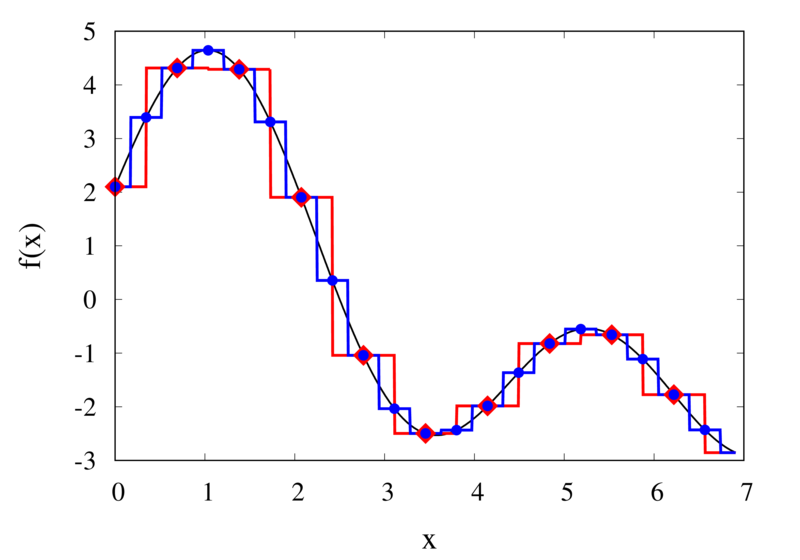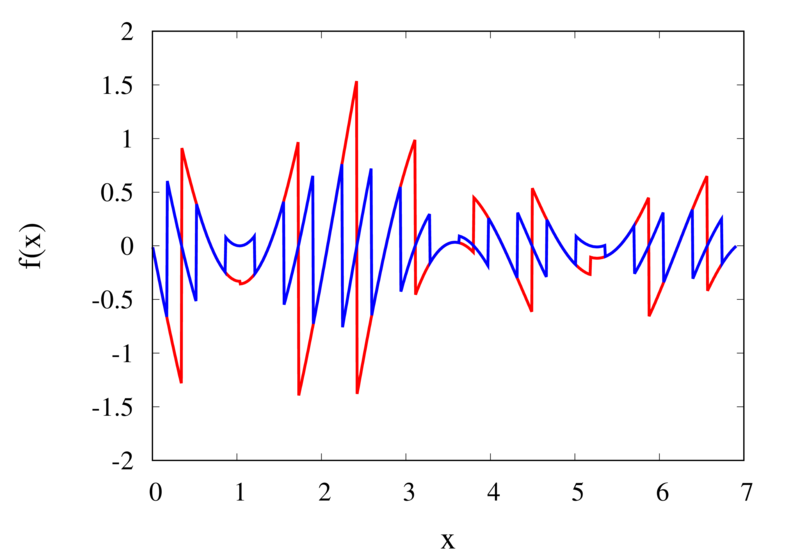Piecewise constant interpolation of a function (left) and the error (right)

The left plot in the figure above shows some smooth function in black and a number of sample points. The case where 10 sample points are taken is shown by the diamonds and the case for 20 sample points is shown by the circles. Also shown are the nearest neighbour interpolations for these two cases. The red curve shows the interpolated function for 10 samples and the blue curve is for the case of 20 samples. The right plot in the figure shows the difference between the original function and the interpolations. Again, the red curve is for the case of 10 samples and the blue curve is for the case of 20 samples. We can see that the piecewise constant interpolation is crude and the errors are quite large.

As expected, the error is smaller when the number of samples is increased. To analyse exactly how big the error is, consider the residual for the zero-order interpolation $R_0(x) = f(x) – p_0(x) = f(x) – f(x_i).$
The first step to analyse the magnitude of the residual is to perform a Taylor expansion of the residual around the point $$x_i$$. We only need the zero order term. Using Taylor’s Theorem and the Cauchy form of the remainder, one can write $R_0(x) = \left[ f(x_i) + f'(\xi_c)(x – x_i)\right] – f(x_i).$
The term in the brackets is the Taylor expansion of $$f(x)$$, and $$\xi_c$$ is some value that lies between $$x_i$$ and $$x$$ and depends on the value of $$x$$. Let’s define the distance between two samples with $$h=x_{i+1}-x_i$$. Assume for the moment that all samples are equidistant. It is not difficult to generalise the arguments for the case when the support points are not equidistant. This means, the maximum value of $$x – x_i$$ is half of the distance between two samples, i.e. $x – x_i \le \frac{h}{2}.$
It os also clear that $$f'(\xi_c) \le |f'(x)|_{\mathrm{max}}$$, where the maximum is over the interval $$|x-x_i| \le h/2$$. The final result for an estimate of the residual error is $|R_0(x)| \le\frac{h}{2} |f'(x)|_{\mathrm{max}}$

#### Linear Interpolation

As we saw above, the piecewise interpolation is easy to implement but the errors can be quite large. Most of the time, linear interpolation is a much better alternative. For functions of a single argument, as we are considering here, the computational expense is not much higher than the piecewise interpolation but the resulting accuracy is much better. Given a location $$x$$, first find $$i$$ such that $x_i \le x < x_{i+1}.$
Then the linear interpolation function $$p_1$$ can be defined as $p_1(x) = \frac{x_{i+1} – x}{x_{i+1} – x_i} f(x_i) + \frac{x – x_i}{x_{i+1} – x_i} f(x_{i+1}).$
The function $$p_1$$ at a point $$x$$ can be viewed as a weighted average of the original function values at the neighbouring points $$x_i$$ and $$x_{i+1}$$. It can be easily seen that $$p(x_i) = f(x_i)$$ for all $$i$$, i.e. the interpolation goes through the sample points exactly.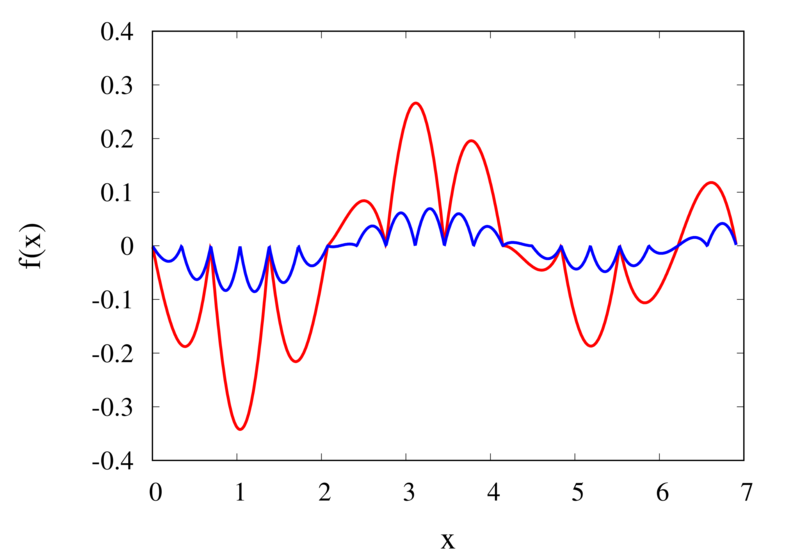Linear interpolation of a function (left) and the error (right)

The left plot in the figure above shows the same function $$f(x)$$ as the figure in the previous section but now together with the linear interpolations for 10 samples (red curve) and 20 samples (blue curve). One can immediately see that the linear interpolation resembles the original function much more closely. The right plot shows the error for the two interpolations. The error is much smaller when compared to the error for the piecewise interpolation. For the 10 sample interpolation, the maximum absolute error of the linear interpolation is about 0.45 compared to a value of over 1.5 for the nearest neighbour interpolation. What’s more, going from 10 to 20 samples improves the error substantially.

One can again try to quantify the error of the linear approximation using Taylor’s Theorem. The first step is to use the Mean Value Theorem that states that there is a point $$x_c$$ between $$x_i$$ and $$x_{i+1}$$ that satisfies $f'(x_c) = \frac{ f(x_{i+1}) – f(x_i) }{ x_{i+1} – x_i }.$
Consider now the error of the linear approximation, $R_1(x) = f(x) – p_1(x) = f(x) – \left[\frac{x_{i+1} – x}{x_{i+1} – x_i} f(x_i) + \frac{x – x_i}{x_{i+1} – x_i} f(x_{i+1})\right].$
The derivative of the error is $R’_1(x) = f'(x) – \frac{ f(x_{i+1}) – f(x_i) }{ x_{i+1} – x_i }.$
The Mean Value Theorem implies that the derivative of the error at $$x_c$$ is zero and the error is at its maximum at that point. In other words, to estimate the maximum error, we only need to find an upper bound of $$|R(x_c)|$$.

We now perform a Taylor expansion of the error around $$x_c$$. Using again the Cauchy form of the remainder, we find $R(x) = R(x_c) + xR'(x_c) + \frac{1}{2}R’^\prime(\xi_c)(x-\xi_c)(x-x_c).$
The second term on the right hand side is zero by construction, and we have $R(x) = R(x_c) + \frac{1}{2}R’^\prime(\xi_c)(x-\xi_c)(x-x_c).$
Let $$h$$ again denote the distance between the two points, $$h=x_{i+1} – x_i$$. We assume that $$x_c – x_i < h/2$$ and use the equation above to calculate $$R(x_i)$$ which we know is zero. If $$x_c$$ was closer to $$x_{i+1}$$ we would have to calculate $$R(x_{i+1})$$ but otherwise the argument would remain the same. So, $R(x_i) = 0 = R(x_c) + \frac{1}{2}R’^\prime(\xi_c)(x_i-\xi_c)(x_i-x_c)$
from which we get $|R(x_c)| = \frac{1}{2}|R’^\prime(\xi_c)(x_i-\xi_c)(x_i-x_c)|.$
To get an upper estimate of the remainder that does not depend on $$x_c$$ or $$\xi_c$$ we can use the fact that both $$x_i-\xi_c \le h/2$$ and $$x_i-x_c \le h/2$$. We also know that $$|R(x)| \le |R(x_c)|$$ over the interval from $$x_i$$ to $$x_{i+1}$$ and $$|R’^\prime(\xi_c)| = |f’^\prime(\xi_c)| \le |f’^\prime(x)|_{\mathrm{max}}$$. Given all this, we end up with $|R(x)| \le \frac{h^2}{8}|f’^\prime(x)|_{\mathrm{max}}.$

The error of the linear interpolation scales with $$h^2$$, in contrast to $$h$$ for the piecewise constant interpolation. This means that increasing the number of samples gives us much more profit in terms of accuracy. Linear interpolation is often the method of choice because of its relative simplicity combined with reasonable accuracy. In a future post, I will be looking at higher-order interpolations. These higher-order schemes will scale even better with the number of samples but this improvement comes at a cost. We will see that the price to be paid is not only a higher computational expense but also the introduction of spurious oscillations that are not present in the original data.

#### Computational Physics: Truncation and Rounding Errors

In a previous post, I talked about accuracy and precision in numerical calculations. Ideally one would like to perform calculations that are perfect in these two aspects. However, this is almost never possible in practical situations. The reduction of accuracy or precision is due to two numerical errors. These errors can be classified into two main groups, round-off errors and truncation errors.

#### Rounding Error

Round-off errors occur due to the limits of numerical precision at which numbers are stored in the computer. As I discussed here a 32-bit floating-point number for example can only store up to 7 or 8 decimal digits. Not just the final result but every intermediate result of a calculation will be rounded to this precision. In some cases, this can result in a much lower precision of the final result. One instance where round-off errors can become a problem happens when the result of a calculation is given by the difference of two large numbers.

#### Truncation Error

Truncation errors occur because of approximations the numerical scheme makes with respect to the underlying model. The name truncation error stems from the fact that in most schemes the underlying model is first expressed as an infinite series which is then truncated allowing it to be calculated on a computer.

### Example: Approximating Pi

Let’s start with a simple task. Use a series to approximate the value of $$\pi$$.

#### Naive summation

One of the traditional ways of calculating $$\pi$$ is by using the $$\arctan$$ function together with the identity $\arctan(1) = \frac{\pi}{4}.$
One can expand $$\arctan$$ into its Taylor series, $\arctan(x) = x – \frac{x^3}{3} +\frac{x^5}{5} – \frac{x^7}{7} + \ldots = \sum_{n=0}^\infty \frac{(-1)^n x^{2n+1}}{2n+1}.$
The terms of the series become increasingly smaller and you could try to add up all the terms up to some maximum $$N$$ in the hope that the remaining infinite sum is small and can be neglected. Inserting $$x=1$$ into the sum will give you an approximation for $$\pi$$, $\pi \approx 4\sum_{n=0}^N \frac{(-1)^n }{2n+1}.$

Here are e implementations for this approximation in C++, Python and JavaScript.

C++

double pi_summation_slow(int N) {
double  sum = 0.0;
int  sign = 1;

for (int  i=0; i<N; ++i) {
sum += sign/(2*i + 1.0);
sign = -sign;
}

return 4*sum;
}

Python

def  pi_summation_slow(N):
sum  =  0.0
sign  =  1

for  i  in  range(0,N):
sum   =  sum  +  sign/(2*i  +  1.0)
sign  =  -sign
return  4*sum

JavaScript

function pi_summation_slow(N) {
let sum = 0.0;
let sign = 1;

for (let i=0; i<N; ++i) {
sum += sign/(2*i + 1.0);
sign = -sign;
}

return 4*sum;
}

Let’s call this function with $$N=10$$. All the results I am showing here are calculated using a Python implementation. We get a result of around 3.0418. The relative error is 0.0318 and is, of course, unacceptable. This error falls under the category of truncation errors because it is caused by not summing up enough terms of the Taylor series. Calling the function with $$N=1000$$ gives us a result of 3.14059 with a relative error of $$3.183\times 10^{-4}$$. The error has improved but is still far off from the possible $$10^{-14}$$ to $$10^{-15}$$ achievable in double-precision arithmetic. The figure below shows how the relative error decreases with the number of iterations.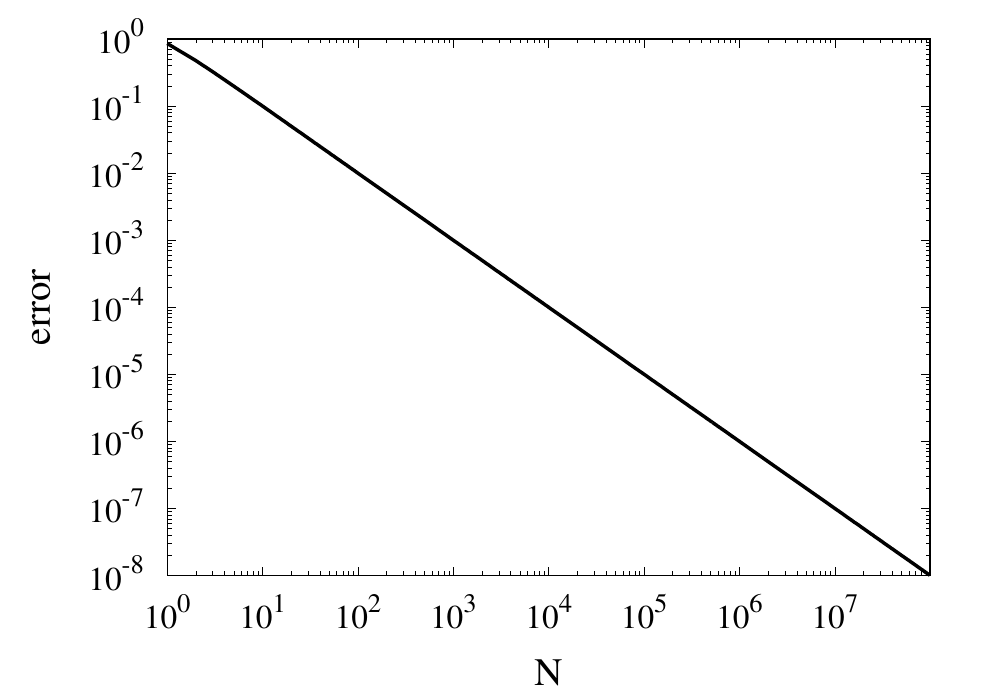Relative error of the simple approximation of $$\pi$$ depending on the number of iterations

From this curve, one h long m wl hat the error decreases with $$1/N$$. If one extrapolates the curve, one finds that it would take $$10^{14}$$ iterations to reach an error below $$10^{-14}$$. Even if this was computationally feasible, the round-off errors of such a long sum would eventually prevent the error from being lowered to this limit.

#### Improvements using Machin’s formula

The technique of calculating $$\pi$$ can be improved in two ways. Firstly, instead of using the Taylor series, you can use Euler’s series for the $$\arctan$$ function.

$\arctan(x) = \sum_{n=0}^\infty \frac{2^{2n} (n!)^2}{(2n + 1)!} \frac{x^{2n + 1}}{(1 + x^2)^{n + 1}}.$

This series converges much more quickly than the Taylor series. The other way to improve convergence is to use trigonometric identities to come up with formulas that converge more quickly. One of the classic equations is the Machin formula for $$\pi$$, first discovered by John Machin in 1706, $\frac{\pi}{4} = 4 \arctan \frac{1}{5} – \arctan \frac{1}{239}$
Here are the implementations for this formula.

C++

double pi_summation_fast(int order) {
using boost::math::factorial;
double sum = 0.0;

for (unsigned int n=0; n<order; ++n) {
double f = factorial<double>(n);
double common = pow(2.0, 2*n)*f*f/factorial<double>(2*n + 1);
double A = pow(25./26., n+1)/pow(5., 2*n+1);
double B = pow(239.*239. / (239.*239. + 1.), n+1)/pow(239., 2*n+1);
sum += common*( 4*A - B );
}

return 4*sum;
}

Python

def pi_summation_fast(N):
sum = 0.0

for n in range(0,N):
f = factorial(n)
common = math.pow(2.0, 2*n)*f*f/factorial(2*n + 1)
A = math.pow(25/26, n+1)/math.pow(5, 2*n+1)
B = math.pow(239*239 / (239*239 + 1), n+1)/math.pow(239, 2*n+1)
sum = sum + common*( 4*A - B )

return 4*sum;

JavaScript

function pi_summation_fast(N) {
let sum = 0.0;

for (let n=0; n<N; ++n) {
const f = factorial(n);
const common = Math.pow(2.0, 2*n)*f*f/factorial(2*n + 1);
const A = pow(25/26, n+1)/pow(5, 2*n+1);
const B = pow(239*239 / (239*239 + 1), n+1)/pow(239, 2*n+1);
sum += common*( 4*A - B );
}

return 4*sum;
}

The table below shows the computed values for $$\pi$$ together with the relative error. You can see that each iteration reduces the error by more than an order of magnitude and only a few iterations are necessary to achieve machine precision accuracy.

N $$S_N$$ error
1 3.060186968243409 0.02591223443732105
2 3.139082236428362 0.0007990906009289966
3 3.141509789149037 2.6376570705797483e-05
4 3.141589818359699 9.024817686074192e-07
5 3.141592554401089 3.157274505454055e-08
6 3.141592650066872 1.1213806035463463e-09
7 3.1415926534632903 4.0267094489200705e-11
8 3.1415926535852132 1.4578249079970333e-12
9 3.1415926535896266 5.3009244691058615e-14
10 3.1415926535897873 1.8376538159566985e-15
11 3.141592653589793 0.0

### Example: Calculating sin(x)

Calculate the value of $$\sin(x)$$ using it’s Taylor series around x=0.

The Taylor series for $$\sin(x)$$ is $\sin x = \sum_{n=0}^\infty \frac{(-1)^n}{(2n+1)!}x^{2n+1}.$
this series is much more well-behaved than the Taylor series for $$\arctan$$ we saw above. Because of the factorial in the denominator, the individual terms of this series will converge reasonably quickly. Here are some naive implementations of this function where the infinite sum has been replaced by a sum from zero to $$N$$.

C++

double taylor_sin(double x, int order)
{
using boost::math::factorial;
double sum = 0.0;
int sign = 1;

for (unsigned int n=0; n<order; ++n)
{
sum += sign*pow(x, 2*n + 1)/factorial<double>(2*n +1);
sign = -sign;
}

return sum;
}

Python

def taylor_sin(x, N):
sum = 0.0
sign = 1

for n in range(0,N):
sum = sum + sign*math.pow(x, 2*n + 1)/factorial(2*n + 1)
sign = -sign

return sum

JavaScript

function taylor_sin(x, N) {
let sum = 0.0;
let sign = 1;

for (let n=0; n<N; n++) {
sum += sign*pow(x, 2*n + 1)/factorial(2*n +1);
sign = -sign;
}

return sum;
}

A good test for this function is the evaluation of $$\sin(x)$$ at values $$x = k\pi$$, where $$k$$ is an integer. We know that $$\sin(k\pi) = 0$$ and the return value from the numeric function can directly be used as the absolute error of the computation. The figure below shows results for some values of $$k$$ plotted against $$N$$.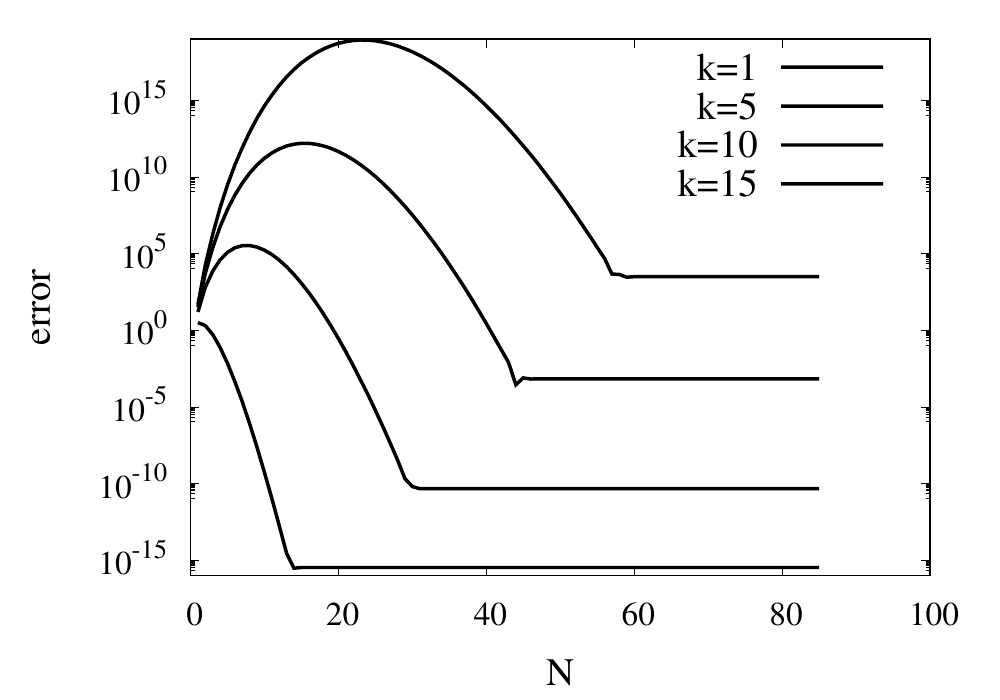For small values of $$k$$, this series converges relatively quickly. But for larger $$k$$ you can see that more and more terms are needed. The error even grows first before being reduced. Just like the example above, the truncation error requires large values of $$N$$ to reach a good accuracy of the result. In practice, you would not calculate the $$\sin$$ function this way. Instead you would make use of known properties, such as $$\sin(2k\pi + x) = \sin(x)$$ for integer $$k$$, to transform the argument into a range where fast convergence is guaranteed.

However, I would like to continue my analysis of this function because it shows two more interesting pitfalls when performing long sums. First, you will notice that the curves in the figure above show dashed lines for $$N>85$$. This is because the implementation I showed above will actually fail with a range error. The pow function and the factorial both start producing numbers that exceed the valid range of double floating-point numbers. The quotient of the two, on the other hand, remains well-behaved. It is, therefore, better to write the Taylor series using a recursive definition of the terms.

$\sin x = \sum_{n=0}^\infty a_n(x),$
with $a_0 = x$
and $a_{n} = -\frac{x^2}{2n(2n+1)}a_{n-1}$

The implementations are given again below.

C++

double taylor_sin_opt(double x, int order)
{
double sum = x;
double an = x;

for (unsigned int n=1; n<order; ++n)
{
an = -x*x*an/(2*n*(2*n+1));
sum += an;
}

return sum;
}

Python

def taylor_sin_opt(x, N):
sum = x
an = x

for n in range(1,N):
an = -x*x*an/(2*n*(2*n+1))
sum = sum + an

return sum

JavaScript

function taylor_sin_opt(x, N) {
let sum = x;
let an = x;

for (let n=1; n<N; n++) {
an = -x*x*an/(2*n*(2*n+1));
sum += an;
}

return sum;
}

The other takeaway from the graphs of the errors is that they don’t always converge to machine accuracy. The reason for this originates from fact that the initial terms of the sum can be quite large but with opposite signs. They should cancel each other out exactly, but they don’t because of numerical round-off errors.

#### Multi Slit Interference

This week I have been playing around with my electromagnetic wave simulation code MPulse. The code simulates Maxwell’s equations using an algorithm known as finite-difference time-domain, FDTD for short. Here, I am simulating the wave interference pattern behind the screen with n slits. For $n=1$ there is only one slit and no interference at all. The slit is less than the wavelength wide so you can see the wave spreading out at a large angle. When $n>1$ the slits are 5 wavelengths apart. With $n=2$ you have the classical double-slit experiment. At the right side of the simulation box, the well-known interference pattern can be observed. For 3 slits the pattern close to the slits becomes more complicated. It settles down to a stable pattern much further to the right. For numbers 4, 5 and 6 the patterns become more and more interesting. You would expect the development of sharper lines but it turns out that, for these larger numbers, the simulation box just wasn’t big enough to see the final far-field pattern.

MPulse is open-source and is available at https://github.com/holgerschmitz/MPulse.

The code uses MPI parallelization and was run on 24 cores for this simulation.

MPulse is a work in progress and any help from enthusiastic developers is greatly appreciated.

#### Computational Physics Basics: Accuracy and Precision

Problems in physics almost always require us to solve mathematical equations with real-valued solutions, and more often than not we want to find functional dependencies of some quantity of a real-valued domain. Numerical solutions to these problems will only ever be approximations to the exact solutions. When a numerical outcome of the calculation is obtained it is important to be able to quantify to what extent it represents the answer that was sought. Two measures of quality are often used to describe numerical solutions: accuracy and precision. Accuracy tells us how will a result agrees with the true value and precision tells us how reproducible the result is. In the standard use of these terms, accuracy and precision are independent of each other.

#### Accuracy

Accuracy refers to the degree to which the outcome of a calculation or measurement agrees with the true value. The technical definition of accuracy can be a little confusing because it is somewhat different from the everyday use of the word. Consider a measurement that can be carried out many times. A high accuracy implies that, on average, the measured value will be close to the true value. It does not mean that each individual measurement is near the true value. There can be a lot of spread in the measurements. But if we only perform the measurement often enough, we can obtain a reliable outcome.

#### Precision

Precision refers to the degree to which multiple measurements agree with each other. The term precision in this sense is orthogonal to the notion of accuracy. When carrying out a measurement many times high precision implies that the outcomes will have a small spread. The measurements will be reliable in the sense that they are similar. But they don’t necessarily have to reflect the true value of whatever is being measured.

#### Accuracy vs Precision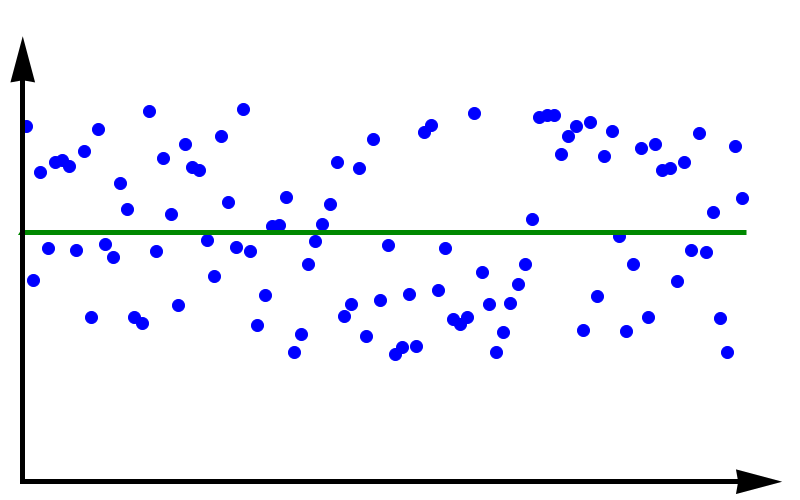Data with high accuracy but low precision. The green line represents the true value.

To fully grasp the concept of accuracy vs precision it is helpful to look at these two plots. The crosses represent measurements whereas the line represents the true value. In the plot above, the measurements are spread out but they all lie around the true value. These measurements can be said to have low precision but high accuracy. In the plot below, all measurements agree with each other, but they do not represent the true value. In this case, we have high precision but low accuracy.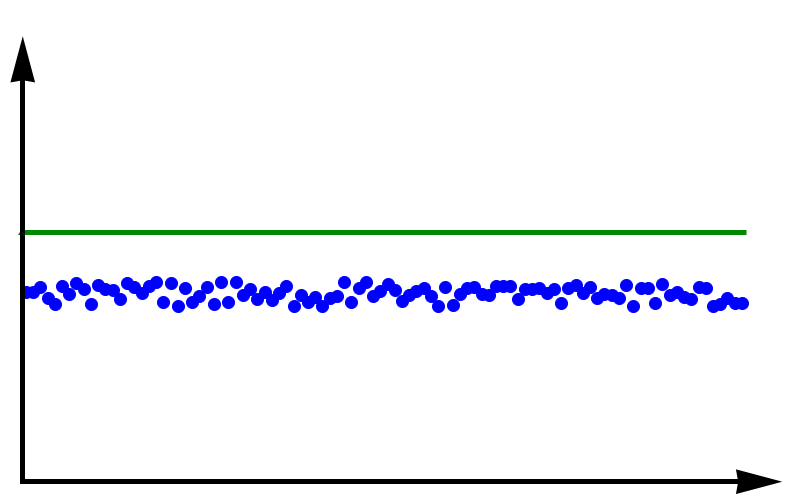Data with high precision but low accuracy. The green line represents the true value.

A moral can be gained from this: just because you always get the same answer doesn’t mean the answer is correct.

When thinking about numerical methods you might object that calculations are deterministic. Therefore the outcome of repeating a calculation will always be the same. But there is a large class of algorithms that are not quite so deterministic. They might depend on an initial guess or even explicitly on some sequence of pseudo-random numbers. In these cases, repeating the calculation with a different guess or random sequence will lead to a different result.

#### Computational Physics Basics: Floating Point Numbers

In a previous contribution, I have shown you that computers are naturally suited to store finite length integer numbers. Most quantities in physics, on the other hand, are real numbers. Computers can store real numbers only with finite precision. Like storing integers, each representation of a real number is stored in a finite number of bits. Two aspects need to be considered. The precision of the stored number is the number of significant decimal places that can be represented. Higher precision means that the error of the representation can be made smaller. Bur precision is not the only aspect that needs consideration. Often, physical quantities can be very large or very small. The electron charge in SI units, for example, is roughly $1.602\times10^{-19}$C. Using a fixed point decimal format to represent this number would require a large number of unnecessary zeros to be stored. Therefore, the range of numbers that can be represented is also important.

In the decimal system, we already have a notation that can capture very large and very small numbers and I have used it to write down the electron charge in the example above. The scientific notation writes a number as a product of a mantissa and a power of 10. The value of the electron charge (without units) is written as
$$1.602\times10^{-19}.$$
Here 1.602 is the mantissa (or the significand) and -19 is the exponent. The general form is
$$m\times 10^n.$$
The mantissa, $m$, will always be between 1 and 10 and the exponent, $n$, has to be chosen accordingly. This format can straight away be translated into the binary system. Here, any number can be written as
$$m\times2^n,$$
with $1\le m<2$. Both $m$ and $n$ can be stored in binary form.

#### Memory layout of floating-point numbers, the IEEE 754 standard

In most modern computers, numbers are stored using 64 bits but some architectures, like smartphones, might only use 32 bits. For a given number of bits, a decision has to be made on how many bits should be used to store the mantissa and how many bits should be used for the exponent. The IEEE 754 standard sets out the memory layout for various size floating-point representations and almost all hardware supports these specifications. The following table shows the number of bits for mantissa and exponent for some IEEE 754 number formats.

Bits Name Sign bit Mantissa bits, m Exponent bits, p Exponent bias Decimal digits
16 half-precision 1 10 5 15 3.31
32 single precision 1 23 8 127 7.22
64 double precision 1 52 11 1023 15.95
128 quadruple precision 1 112 15 16383 34.02

The layout of the bits is as follows. The first, most significant bit represents the sign of the number. A 0 indicates a positive number and a 1 indicates a negative number. The next $p$ bits store the exponent. The exponent is not stored as a signed integer, but as an unsigned integer with offset. This offset, or bias, is chosen to be $2^p – 1$ so that a leading zero followed by all ones corresponds to an exponent of 0.

The remaining bits store the mantissa. The mantissa is always between 1 and less than 2. This means that, in binary, the leading bit is always equal to one and doesn’t need to be stored. The $m$ bits, therefore, only store the fractional part of the mantissa. This allows for one extra bit to improve the precision of the number.

Example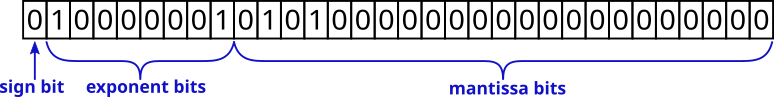The number 5.25 represented by a 32-bit floating-point. In binary, the number is $1.0101\times2^2$. The fractional part of the mantissa is stored in the mantissa bits. The exponent is $127+2$.

#### Infinity and NaN

The IEEE 754 standard defines special numbers that represent infinity and the not-a-number state. Infinity is used to show that a result of a computation has exceeded the allowed range. It can also result from a division by zero. Infinity is represented by the maximum exponent, i.e. all $p$ bits of the exponent are set to 1. In addition, the $m$ bits of the mantissa are set to 0. The sign bit is still used for infinity. This means it is possible to store a +Inf and a -Inf value.

Example

The special state NaN is used to store results that are not defined or can’t otherwise be represented. For example, the operation $\sqrt{-1}$ will result in a not-a-number state. Similar to infinity, it is represented by setting the $p$ exponent bits to 1. To distinguish it from infinity, the mantissa can have any non-zero value.

#### Subnormal Numbers

As stated above, all numbers in the regular range will be represented by a mantissa between 1 and 2 so that the leading bit is always 1. Numbers very close to zero will have a small exponent value. Once the exponent is exactly zero, it is better to explicitly store all bits of the mantissa and allow the first bit to be zero. This allows even smaller numbers to be represented than would otherwise be possible. Extending the range in this way comes at the cost of reduced precision of the stored number.

Example

#### Floating Point Numbers in Python, C++, and JavaScript

Both Python and JavaScript exclusively store floating-point numbers using 64-bit precision. In fact, in JavaScript, all numbers are stored as 64-bit floating-point, even integers. This is the reason for the fact that integers in JavaScript only have 53 bits. They are stored in the mantissa of the 64-bit floating-point number.

C++ offers a choice of different precisions

Type Alternative Name Number of Bits
float single precision usually 32 bits
double double precision usually 64 bits
long double extended precision architecture-dependent,
not IEEE 754,
usually 80 bits

#### Spherical Blast Wave Simulation

Here is another animation that I made using the Vellamo fluid code. It shows two very simple simulations of spherical blast waves. The first simulation has been carried out in two dimensions. The second one shows a very similar setup but in three dimensions.

You might have seen some youtube videos on blast waves and dimensional analysis on the Sixty Symbols channel or on Numberphile. The criterion for the dimensional analysis given in those videos is true for strong blast waves. The simulation that I carried out, looks at the later stage of these waves when the energy peters out and the strong shock is replaced by a compression wave that travels at a constant velocity. You can still see some of the self-similar behaviour of the Sedov-Taylor solution during the very early stages of the explosion. But after the speed of the shock has slowed down to the sound speed, the compression wave continues to travel at the speed of sound, gradually losing its energy.

The video shows the energy density over time. The energy density includes the thermal energy as well as the kinetic energy of the gas.

For those of you who are interested in the maths and the physics, the code simulates the Euler equations of a compressible fluid. These equations are a model for an ideal adiabatic gas. For more information about the Euler equations check out my previous post.

#### Computational Physics Basics: Integers in C++, Python, and JavaScript

In a previous post, I wrote about the way that the computer stores and processes integers. This description referred to the basic architecture of the processor. In this post, I want to talk about how different programming languages present integers to the developer. Programming languages add a layer of abstraction and in different languages that abstraction may be less or more pronounced. The languages I will be considering here are C++, Python, and JavaScript.

### Integers in C++

C++ is a language that is very close to the machine architecture compared to other, more modern languages. The data that C++ operates on is stored in the machine’s memory and C++ has direct access to this memory. This means that the C++ integer types are exact representations of the integer types determined by the processor architecture.

The following integer datatypes exist in C++

Type Alternative Names Number of Bits G++ on Intel 64 bit (default)
char at least 8 8
short int short at least 16 16
int at least 16 32
long int long at least 32 64
long long int long long at least 64 64

This table does not give the exact size of the datatypes because the C++ standard does not specify the sizes but only lower limits. It is also required that the larger types must not use fewer bits than the smaller types. The exact number of bits used is up to the compiler and may also be changed by compiler options. To find out more about the regular integer types you can look at this reference page.

The reason for not specifying exact sizes for datatypes is the fact that C++ code will be compiled down to machine code. If you compile your code on a 16 bit processor the plain int type will naturally be limited to 16 bits. On a 64 bit processor on the other hand, it would not make sense to have this limitation.

Each of these datatypes is signed by default. It is possible to add the signed qualifier before the type name to make it clear that a signed type is being used. The unsigned qualifier creates an unsigned variant of any of the types. Here are some examples of variable declarations.

char c; // typically 8 bit
unsigned int i = 42; // an unsigned integer initialised to 42
signed long l; // the same as "long l" or "long int l"


As stated above, the C++ standard does not specify the exact size of the integer types. This can cause bugs when developing code that should be run on different architectures or compiled with different compilers. To overcome these problems, the C++ standard library defines a number of integer types that have a guaranteed size. The table below gives an overview of these types.

Signed Type Unsigned Type Number of Bits
int8_t uint8_t 8
int16_t uint16_t 16
int32_t uint32_t 32
int64_t uint64_t 64

More details on these and similar types can be found here.

The code below prints a 64 bit int64_t using the binary notation. As the name suggests, the bitset class interprets the memory of the data passed to it as a bitset. The bitset can be written into an output stream and will show up as binary data.

#include  <bitset>

void printBinaryLong(int64_t num) {
std::cout << std::bitset<64>(num) << std::endl;
}


### Integers in Python

Unlike C++, Python hides the underlying architecture of the machine. In order to discuss integers in Python, we first have to make clear which version of Python we are talking about. Python 2 and Python 3 handle integers in a different way. The Python interpreter itself is written in C which can be regarded in many ways as a subset of C++. In Python 2, the integer type was a direct reflection of the long int type in C. This meant that integers could be either 32 or 64 bit, depending on which machine a program was running on.

This machine dependence was considered bad design and was replaced be a more machine independent datatype in Python 3. Python 3 integers are quite complex data structures that allow storage of arbitrary size numbers but also contain optimizations for smaller numbers.

It is not strictly necessary to understand how Python 3 integers are stored internally to work with Python but in some cases it can be useful to have knowledge about the underlying complexities that are involved. For a small range of integers, ranging from -5 to 256, integer objects are pre-allocated. This means that, an assignment such as

n = 25

will not create the number 25 in memory. Instead, the variable n is made to reference a pre-allocated piece of memory that already contained the number 25. Consider now a statement that might appear at some other place in the program.

a = 12
b = a + 13

The value of b is clearly 25 but this number is not stored separately. After these lines b will reference the exact same memory address that n was referencing earlier. For numbers outside this range, Python 3 will allocate memory for each integer variable separately.

Larger integers are stored in arbitrary length arrays of the C int type. This type can be either 16 or 32 bits long but Python only uses either 15 or 30 bits of each of these "digits". In the following, 32 bit ints are assumed but everything can be easily translated to 16 bit.

Numbers between −(230 − 1) and 230 − 1 are stored in a single int. Negative numbers are not stored as two’s complement. Instead the sign of the number is stored separately. All mathematical operations on numbers in this range can be carried out in the same way as on regular machine integers. For larger numbers, multiple 30 bit digits are needed. Mathamatical operations on these large integers operate digit by digit. In this case, the unused bits in each digit come in handy as carry values.

### Integers in JavaScript

Compared to most other high level languages JavaScript stands out in how it deals with integers. At a low level, JavaScript does not store integers at all. Instead, it stores all numbers in floating point format. I will discuss the details of the floating point format in a future post. When using a number in an integer context, JavaScript allows exact integer representation of a number up to 53 bit integer. Any integer larger than 53 bits will suffer from rounding errors because of its internal representation.

const a = 25;
const b = a / 2;

In this example, a will have a value of 25. Unlike C++, JavaScript does not perform integer divisions. This means the value stored in b will be 12.5.

JavaScript allows bitwise operations only on 32 bit integers. When a bitwise operation is performed on a number JavaScript first converts the floating point number to a 32 bit signed integer using two’s complement. The result of the operation is subsequently converted back to a floating point format before being stored.